edHelper subscribers - Create a new printable

Number of Pages
 Select the number of pages: 1 page 2 pages 3 pages 4 pages

Include an answer key (answer keys will be at the end of the printable)

Sample edHelper.com - Math Review Worksheet

 Name _____________________________Date ___________________
Algebra
Simplify.
1.
 5-7 × 59

2.
 611 × 612

3.
 2-7 × 2-6

Solve each inequality.
4.
 157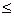q  -
 195

5.
-2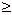d  +
 32

6.
 35
+  b23

Factor completely.
 7 x2 - 9x + 20
 8 x2 + 5x - 24
 9 x2 + x - 132

 10 (4x + 19) + (16x + 10)
 11 (20x + 12) + (20x + 6)
 12 (6x2 + 18x + 20) + (9x2 + 11x + 5)

Is the given expression a factor of the polynomial?
 13 f(x) = 2x3 - 24x2 + 72x  Is x - 6 a factor?
 14 f(x) = x3 - 64  Is x - 4 a factor?
 15 f(x) = -x3 - 3x2 + 70x  Is x + 10 a factor?

Complete.
16.   Solve for c:
 4c5
-
 12
=
 -252

17.   Simplify:
6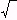192
+
512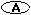29677125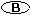204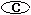586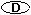16826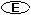84112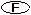583

Use the quadratic formula to solve each equation.
 18 6x2 + 5x - 3 = 0
19.
 -9x2 - x + 11 = 0

20.
 -4x2 + 8x + 18 = -8x2 + 24

Complete.
 21 There are three consecutive multiples of nine. The sum of the first two numbers is 2,853. What are the integers?
 22 Taylor leases cars for \$79 per month plus two cents per mile the car is driven up to 72 miles and ten cents per mile over 72 miles. Rachel and Kaitlyn both rented a car from Taylor. In February, Rachel paid \$17.82 more than Kaitlyn. How many more miles did Rachel drive?

Sample
This is only a sample worksheet.

edHelper subscribers - Create a new printable

Number of Pages
 Select the number of pages: 1 page 2 pages 3 pages 4 pages